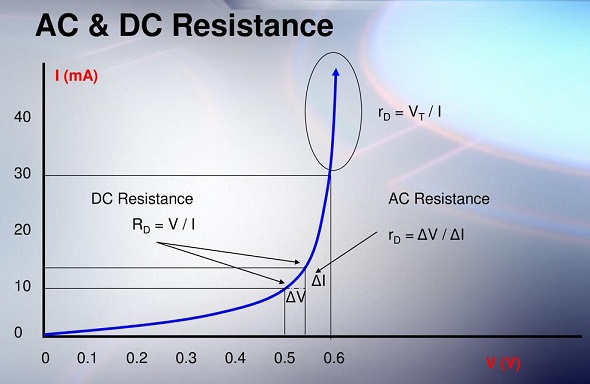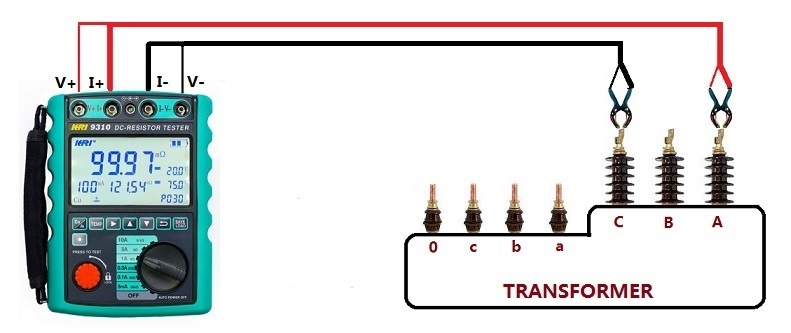Technology Literature

# What is the difference between DC& AC resistance and how to measure it?

What is DC resistance?

The DC resistance is the resistance shown by the component when it is connected to DC, that is, the inherent static resistance of the component. For example, when a coil is connected to direct current and alternating current, its resistance is different. When connected to alternating current, in addition to direct current resistance, the coil also has reactance, which reflects the cooperative effect of resistance and reactance, called impedance.

The unit of resistance is “Ohm” and it is denoted by and it is represented by “R”.

What is the difference between DC resistance and AC resistance?
1. For linear circuits, that is, no matter how much voltage and current are applied to the resistor, the resistance value remains unchanged. There is no such thing as AC resistance here.

2. For nonlinear resistors: the resistance value varies with the applied voltage (current) value, or the relationship between voltage and current is not a straight line. For example: the input characteristics of the triode, that is, the resistance between the b and e poles, is not a fixed value. Base current and base voltage are not linear. At a certain Ib value, corresponding to the base voltage Ub, the DC resistance at this point is Ub/Ib. The AC resistance at this point is the slope value of the tangent line at this point on the input characteristic curve = (△ub/△ib). AC resistance is not the same as DC resistance. On the output characteristic curve of the triode, it is similar. At a certain operating point, the corresponding Uc/Ic is the DC resistance at that point, while the AC resistance is very large, because the change of Uc has little effect on Ic, and the value of (△uc/△ic) is very large.How to Test AC Resistance:

In AC Circuits (Capacitive or inductive Load), Resistance = Impedance i.e., R = Z

Z = √ (R2 + XL2)… In case of Inductive Load

Z = √ (R2 + XC2)…In case of Capacitive Load

Z = √ (R2 + (XL– XC)2…In case of both inductive and capacitive Loads.

XL = Inductive reactance

XL = 2πfL…Where L = Inductance in Henry

And;

XC = Capacitive reactance

XC = 1/2πfC… Where C = Capacitance in Farads.

How to Test DC Resistance?
It is generally tested directly by the instrument. DC resistance measuring instrument, DC resistance tester, transformer DC resistance tester, DC resistance detector, DC digital bridge, etc.
A typical four-wire measurement method is used. In order to improve the accuracy of measuring resistance (especially low resistance). The program-controlled constant current source, the program-controlled preamplifier, and the A/D converter constitute the main body of the measurement circuit.

The central control unit applies a constant and high-precision current to the external load under test by controlling the constant current source, and then processes the obtained data (including test voltage, current test current, etc.) to obtain the actual resistance value.